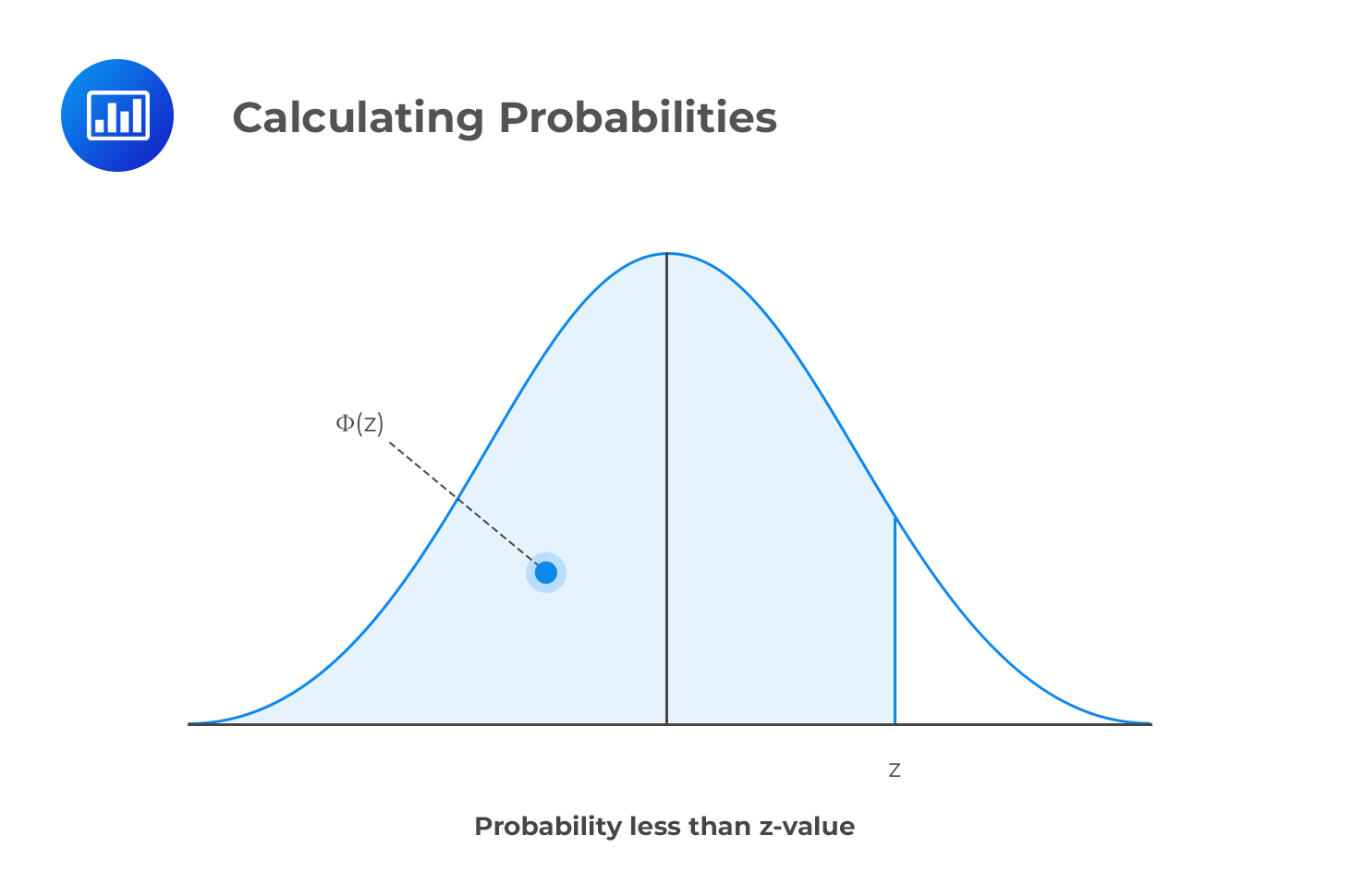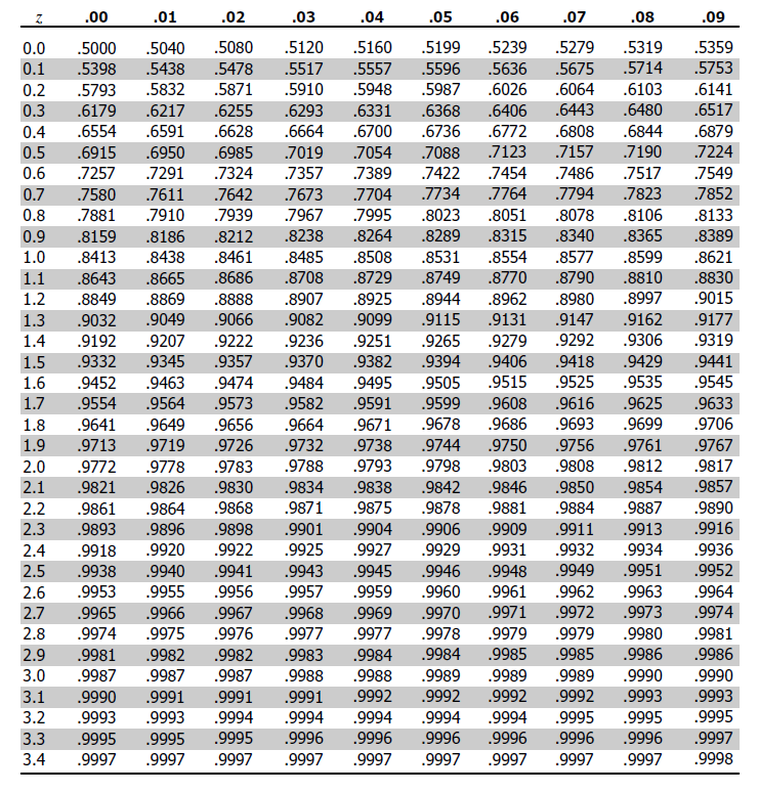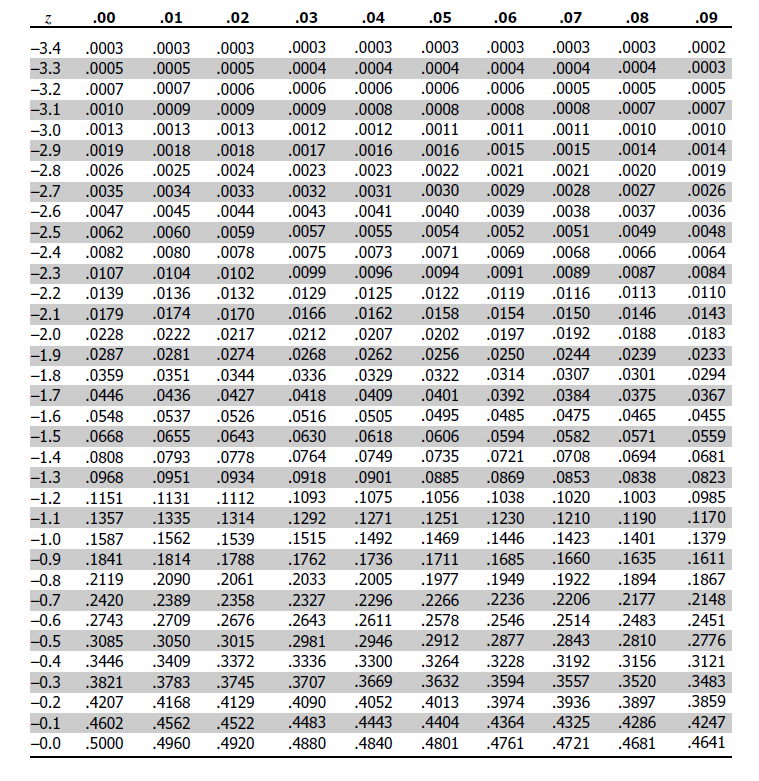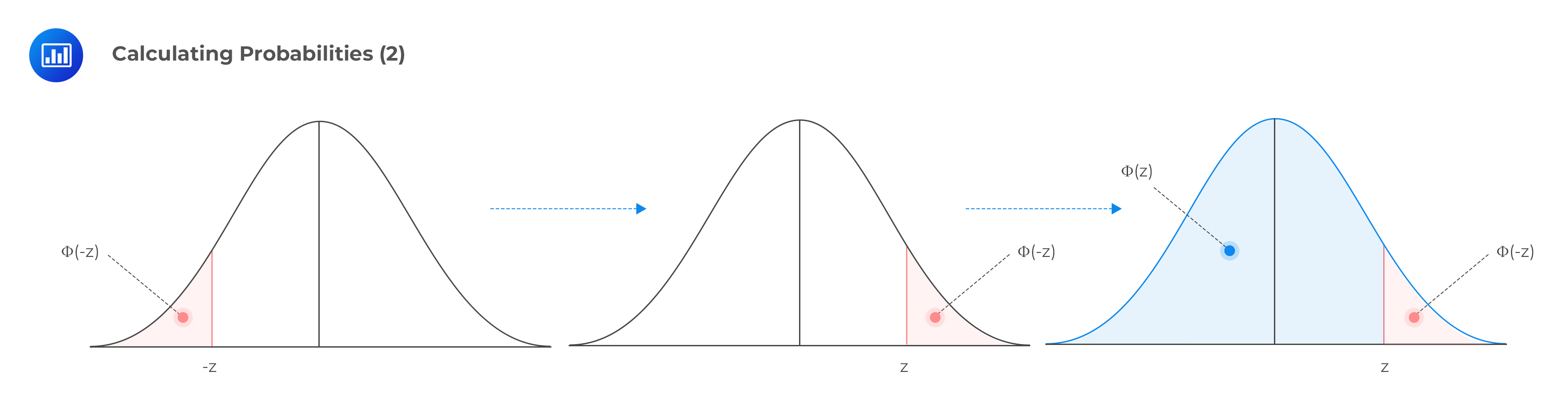Limited Time Offer: Save 10% on all 2021 and 2022 Premium Study Packages with promo code: BLOG10    Select your Premium Package »Using the Standard Normal Distribution to Calculate Probabilities

Using the standard normal distribution table, we can confirm that a normally distributed random variable $$Z$$, with a mean equal to 0 and variance equal to 1, is less than or equal to $$z$$, i.e., $$P(Z ≤ z)$$.

However, the table does this only when we have positive values of $$z$$. Simply put, if an examiner asks you to find the probability behind a given positive z-value, you will have to look it up directly on the table knowing that $$P(Z ≤ z) = θ(z)$$ when $$z$$ is positive.Positive z-values

Example: Using the z-score Table

The returns on ABC stock are normally distributed where the mean is $0.60 with a standard deviation of$0.20. The probability that the return is less than $1 is closest to: Solution If the return is$0.10, then $$x = 0.1$$ (this is our observed value). Therefore,

\begin{align} z &=\frac{x-\mu}{\sigma}\\&=\frac{1-0.6}{0.2}\\ &=2\ \text{(The return of \1 is two and a half standard deviations below the mean)}\end{align}

First, you’d be required to calculate the z-value (2 in this case). $$P(Z ≤ 1)$$ can be read off directly from the table

You just move down and locate the z-value that lies to the right of “2,” i.e., 0.9772.Negative z-values

If we have a negative z-value and do not have access to the negative values from the table (as shown below), we can still calculate the corresponding probability by noting that:

\begin{align} P(Z \le -z) & = 1 – P(Z \le z) \\ \theta(–z) & = 1 – \theta(z) \\ 0.0228 & = 1 – 0.9772 \end{align}This relationship is true when we consider the following facts:

1. The total area (probability) under the standard normal distribution is 1.
2. The standardized normal distribution is symmetrical about the mean.Question

Calculate P(Z  ≤ -2.5).

A. 0.9938

B. 0.0062

C. 0.06

Solution

\begin{align*} P(Z \le -2.5) & = 1 – P(Z \le 2.5) \\ & = 1 – 0.9938 \\ & = 0.0062 \\ \end{align*}

Featured Study with UsCFA® Exam and FRM® Exam Prep Platform offered by AnalystPrep

Study Platform

Learn with Us

Subscribe to our newsletter and keep up with the latest and greatest tips for success
Online TutoringOur videos feature professional educators presenting in-depth explanations of all topics introduced in the curriculum.

Video LessonsSergio Torrico
2021-07-23
Excelente para el FRM 2 Escribo esta revisión en español para los hispanohablantes, soy de Bolivia, y utilicé AnalystPrep para dudas y consultas sobre mi preparación para el FRM nivel 2 (lo tomé una sola vez y aprobé muy bien), siempre tuve un soporte claro, directo y rápido, el material sale rápido cuando hay cambios en el temario de GARP, y los ejercicios y exámenes son muy útiles para practicar.diana
2021-07-17
So helpful. I have been using the videos to prepare for the CFA Level II exam. The videos signpost the reading contents, explain the concepts and provide additional context for specific concepts. The fun light-hearted analogies are also a welcome break to some very dry content. I usually watch the videos before going into more in-depth reading and they are a good way to avoid being overwhelmed by the sheer volume of content when you look at the readings.Kriti Dhawan
2021-07-16
A great curriculum provider. James sir explains the concept so well that rather than memorising it, you tend to intuitively understand and absorb them. Thank you ! Grateful I saw this at the right time for my CFA prep.nikhil kumar
2021-06-28
Very well explained and gives a great insight about topics in a very short time. Glad to have found Professor Forjan's lectures.Marwan
2021-06-22
Great support throughout the course by the team, did not feel neglectedBenjamin anonymous
2021-05-10
I loved using AnalystPrep for FRM. QBank is huge, videos are great. Would recommend to a friendDaniel Glyn
2021-03-24
I have finished my FRM1 thanks to AnalystPrep. And now using AnalystPrep for my FRM2 preparation. Professor Forjan is brilliant. He gives such good explanations and analogies. And more than anything makes learning fun. A big thank you to Analystprep and Professor Forjan. 5 stars all the way!michael walshe
2021-03-18
Professor James' videos are excellent for understanding the underlying theories behind financial engineering / financial analysis. The AnalystPrep videos were better than any of the others that I searched through on YouTube for providing a clear explanation of some concepts, such as Portfolio theory, CAPM, and Arbitrage Pricing theory. Watching these cleared up many of the unclarities I had in my head. Highly recommended.